Related Articles
Data visualization with different Charts in Python
• Difficulty Level : Easy
• Last Updated : 09 Mar, 2018

Data Visualization is the presentation of data in graphical format. It helps people understand the significance of data by summarizing and presenting huge amount of data in a simple and easy-to-understand format and helps communicate information clearly and effectively.

Consider this given Data-set for which we will be plotting different charts :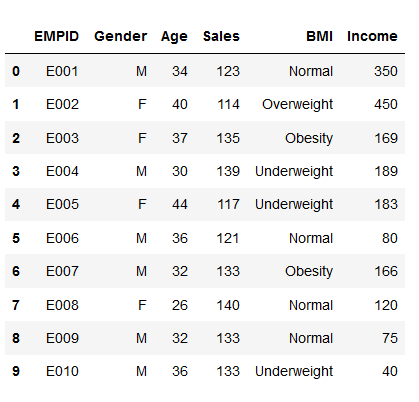Different Types of Charts for Analyzing & Presenting Data

1. Histogram :
The histogram represents the frequency of occurrence of specific phenomena which lie within a specific range of values and arranged in consecutive and fixed intervals.

In below code histogram is plotted for `Age, Income, Sales`. So these plots in the output shows frequency of each unique value for each attribute.

 `# import pandas and matplotlib ` `import` `pandas as pd ` `import` `matplotlib.pyplot as plt ` ` `  `# create 2D array of table given above ` `data ``=` `[[``'E001'``, ``'M'``, ``34``, ``123``, ``'Normal'``, ``350``], ` `        ``[``'E002'``, ``'F'``, ``40``, ``114``, ``'Overweight'``, ``450``], ` `        ``[``'E003'``, ``'F'``, ``37``, ``135``, ``'Obesity'``, ``169``], ` `        ``[``'E004'``, ``'M'``, ``30``, ``139``, ``'Underweight'``, ``189``], ` `        ``[``'E005'``, ``'F'``, ``44``, ``117``, ``'Underweight'``, ``183``], ` `        ``[``'E006'``, ``'M'``, ``36``, ``121``, ``'Normal'``, ``80``], ` `        ``[``'E007'``, ``'M'``, ``32``, ``133``, ``'Obesity'``, ``166``], ` `        ``[``'E008'``, ``'F'``, ``26``, ``140``, ``'Normal'``, ``120``], ` `        ``[``'E009'``, ``'M'``, ``32``, ``133``, ``'Normal'``, ``75``], ` `        ``[``'E010'``, ``'M'``, ``36``, ``133``, ``'Underweight'``, ``40``] ] ` ` `  `# dataframe created with ` `# the above data array ` `df ``=` `pd.DataFrame(data, columns ``=` `[``'EMPID'``, ``'Gender'``,  ` `                                    ``'Age'``, ``'Sales'``, ` `                                    ``'BMI'``, ``'Income'``] ) ` ` `  `# create histogram for numeric data ` `df.hist() ` ` `  `# show plot ` `plt.show() `

Output :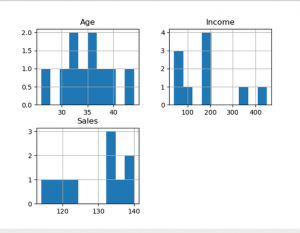2. Column Chart :
A column chart is used to show a comparison among different attributes, or it can show a comparison of items over time.

 `# Dataframe of previous code is used here ` ` `  `# Plot the bar chart for numeric values ` `# a comparison will be shown between ` `# all 3 age, income, sales ` `df.plot.bar() ` ` `  `# plot between 2 attributes ` `plt.bar(df[``'Age'``], df[``'Sales'``]) ` `plt.xlabel(``"Age"``) ` `plt.ylabel(``"Sales"``) ` `plt.show() `

Output :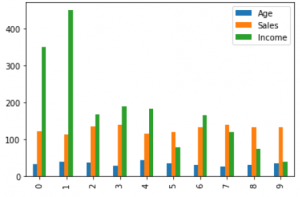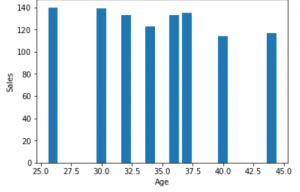3. Box plot chart :
A box plot is a graphical representation of statistical data based on the` minimum, first quartile, median, third quartile, and maximum`. The term “box plot” comes from the fact that the graph looks like a rectangle with lines extending from the top and bottom. Because of the extending lines, this type of graph is sometimes called a box-and-whisker plot. For quantile and median refer to this Quantile and median.

 `# For each numeric attribute of dataframe ` `df.plot.box() ` ` `  `# individual attribute box plot ` `plt.boxplot(df[``'Income'``]) ` `plt.show() `

Output :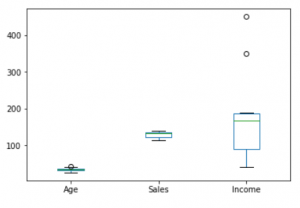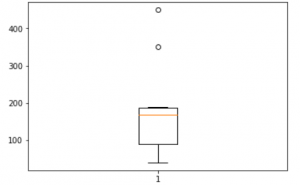4. Pie Chart :
A pie chart shows a static number and how categories represent part of a whole the composition of something. A pie chart represents numbers in percentages, and the total sum of all segments needs to equal 100%.

 `plt.pie(df[``'Age'``], labels ``=` `{``"A"``, ``"B"``, ``"C"``, ` `                             ``"D"``, ``"E"``, ``"F"``, ` `                             ``"G"``, ``"H"``, ``"I"``, ``"J"``}, ` `                              `  `autopct ``=``'% 1.1f %%'``, shadow ``=` `True``) ` `plt.show() ` ` `  `plt.pie(df[``'Income'``], labels ``=` `{``"A"``, ``"B"``, ``"C"``, ` `                                ``"D"``, ``"E"``, ``"F"``, ` `                                ``"G"``, ``"H"``, ``"I"``, ``"J"``}, ` `                                 `  `autopct ``=``'% 1.1f %%'``, shadow ``=` `True``) ` `plt.show() ` ` `  `plt.pie(df[``'Sales'``], labels ``=` `{``"A"``, ``"B"``, ``"C"``, ` `                               ``"D"``, ``"E"``, ``"F"``, ` `                               ``"G"``, ``"H"``, ``"I"``, ``"J"``}, ` `autopct ``=``'% 1.1f %%'``, shadow ``=` `True``) ` `plt.show() `

Output :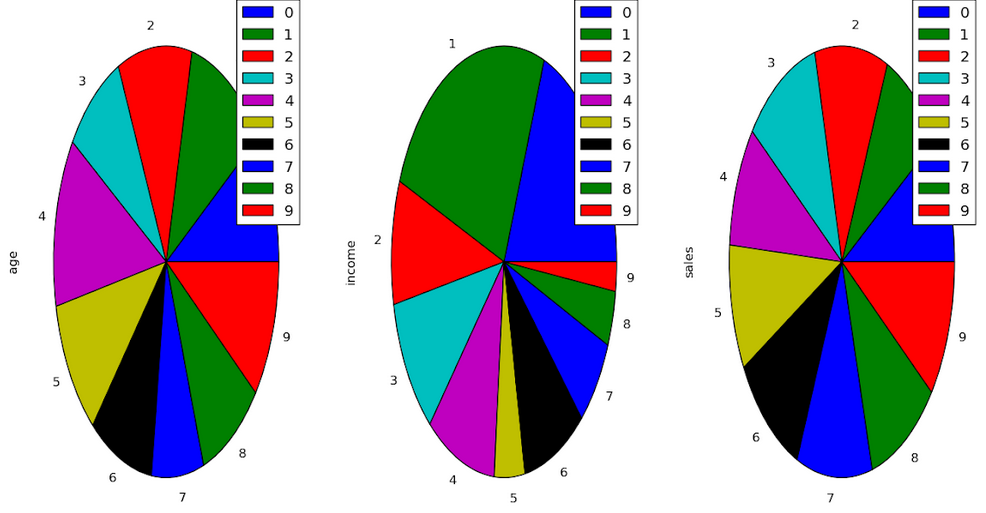5. Scatter plot :
A scatter chart shows the relationship between two different variables and it can reveal the distribution trends. It should be used when there are many different data points, and you want to highlight similarities in the data set. This is useful when looking for outliers and for understanding the distribution of your data.

 `# scatter plot between income and age ` `plt.scatter(df[``'income'``], df[``'age'``]) ` `plt.show() ` ` `  `# scatter plot between income and sales ` `plt.scatter(df[``'income'``], df[``'sales'``]) ` `plt.show() ` ` `  `# scatter plot between sales and age ` `plt.scatter(df[``'sales'``], df[``'age'``]) ` `plt.show() `

Output :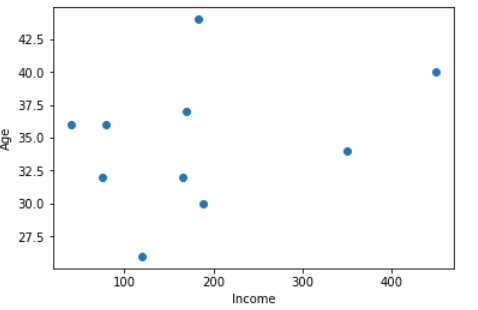Attention geek! Strengthen your foundations with the Python Programming Foundation Course and learn the basics.

To begin with, your interview preparations Enhance your Data Structures concepts with the Python DS Course.

My Personal Notes arrow_drop_up
Recommended Articles
Page :